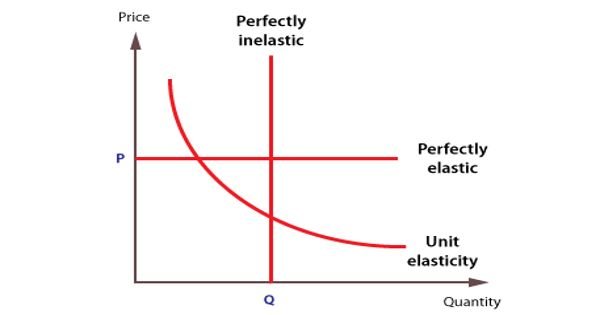Economics

# Price elasticity of demandPrice elasticity of demand is a measurement of the change in consumption of a product in relation to a change in its price. A good’s price elasticity of demand is a measure of how sensitive the quantity demanded of it is to its price. When the price rises, quantity demanded falls for almost any good, but it falls more for some than for others. Demand is price elastic if a change in price leads to a bigger % change in demand; therefore the PED will, therefore, be greater than 1.

Price elasticity of demand measures the responsiveness of demand after a change in a product’s own price. It measures the responsiveness of demand after a change in price.

The price elasticity gives the percentage change in quantity demanded when there is a one percent increase in price, holding everything else constant. It shows the relationship between price and quantity demanded and provides a precise calculation of the effect of a change in price on quantity demanded. If the elasticity is -2, that means a one percent price rise leads to a two percent decline in quantity demanded. Expressed mathematically, it is:

Price Elasticity of Demand = % Change in Quantity Demanded / % Change in Price

Price elasticity of demand is an economic measurement of how the quantity demanded of a good will be affected by changes in its price. Other elasticities measure how the quantity demanded changes with other variables (e.g. the income elasticity of demand for consumer income changes). Economists use price elasticity to understand how supply and demand for a product change when its price changes. When the price elasticity of a good is less than 1, it’s considered inelastic. That means a one-unit increase in price resulted in a less than one unit decrease in demand.

Economists have found that the prices of some goods are very inelastic. Price elasticities are negative except in special cases. That is, a reduction in price does not increase demand much, and an increase in price does not hurt demand either. If a good is said to have an elasticity of 2, it almost always means that the good has an elasticity of -2 according to the formal definition. The phrase “more elastic” means that a good’s elasticity has greater magnitude, ignoring the sign. For example, gasoline has little price elasticity of demand. Drivers will continue to buy as much as they have to, as will airlines, the trucking industry, and nearly every other buyer.

Demand for a good is said to be elastic when the elasticity is greater than one. Theoretically, revenue will be maximized when the price elasticity of a good equals 1, or in other words, when demand is unit elastic. A good with an elasticity of -2 has elastic demand because quantity falls twice as much as the price increase; an elasticity of -0.5 indicates inelastic demand because the quantity response is half the price increase. Pricing, and more specifically your company’s pricing strategy, is the one area applicable to marketing and product that still contains a considerable amount of guesswork.

Information Source: